# The initial diagram has two panels.The left graph depicts a perfectly inelastic demand curve. The right graph depicts a...

The initial diagram has two panels.The left graph depicts a perfectly inelastic demand curve. The right graph depicts a relatively flat sloped demand curve. The initial equilibrium in each graph is labeled point A. We will impose a \$3 per unit tax in the left graph, and a \$4 per unit tax in the right graph.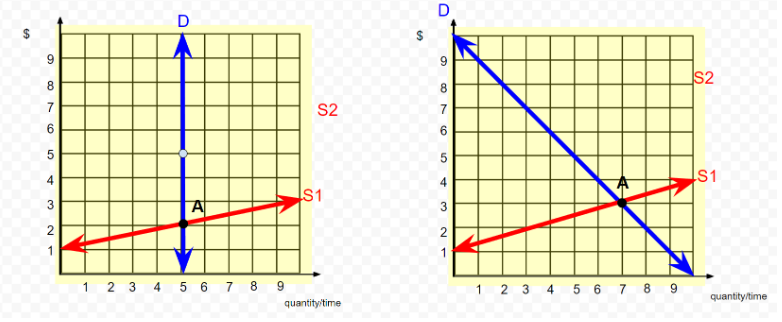Make a copy of the graph, then follow the below instructions to draw the graph:

• 1. In the left graph \$3 above and parallel to the original supply curve, draw a new supply curve, put a black dot at the new equilibrium and label it as point B.

• 2. Draw a vertical arrow between the two supply curves, and label it as a \$3 Tax.

• 3. Shade the rectangle representing the rectangle for the tax burden on the buyer.

• 4 In the right graph \$4 above and parallel to the original supply curve, draw a new supply curve, put a black dot at the new equilibrium and label it as point B.

• 5. Draw a vertical arrow between the two supply curves, and label it as a \$4 Tax.

• 6. Shade the rectangles representing the rectangle for the tax burden on the buyer, and the seller, respectively.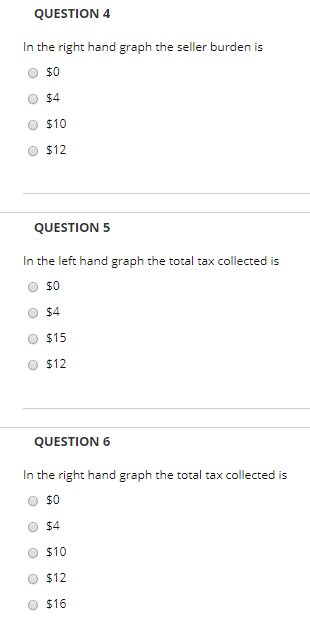S2 S2 S1 8 9quantity/time 1 2 3 4 5 6 7 8 9
QUESTION 4 In the right hand graph the seller burden is O \$0 O \$4 O \$10 O \$12 QUESTION 5 In the left hand graph the total tax collected is O \$0 O \$4 O \$15 O \$12 QUESTION 6 In the right hand graph the total tax colected is O \$0 O \$4 O \$10 O \$12 O \$16

Answer -4 Correct option is \$4.

Producer Burden is 4(3-2) = 4

Total tax collected is 5(5-2) = 15

Total tax collected is 4(6-2) = 16.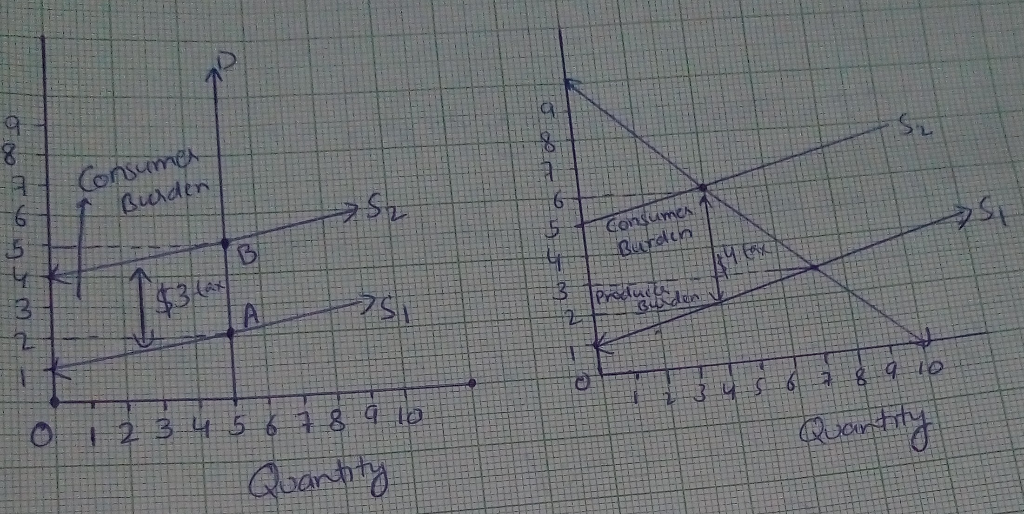##### Add Answer of: The initial diagram has two panels.The left graph depicts a perfectly inelastic demand curve. The right graph depicts a...
Similar Homework Help Questions
• ### Question Number 3 please. 1. Assuming the curves on the right are the actual market Demand...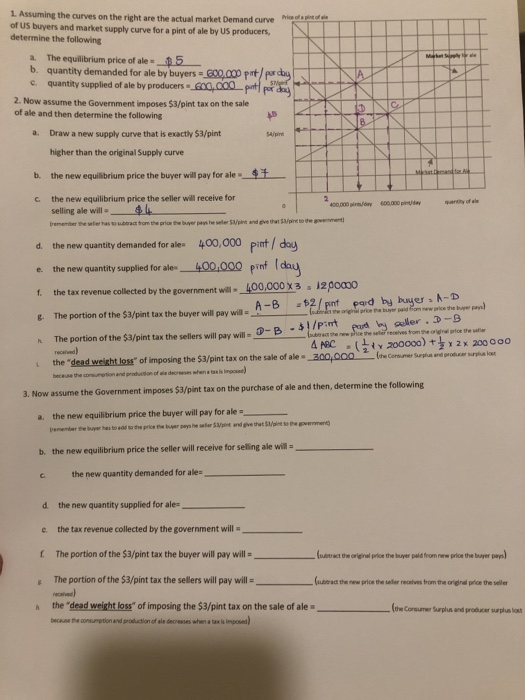Question Number 3 please. 1. Assuming the curves on the right are the actual market Demand curve of US buyers and market supply curve for a pint of ale by US producers, determine the following a. The equilibrium price of ale 0.5 b. quantity demanded for ale by buyers = 600.000 potpurday c. quantity supplied of ale by producers 600,000 potpor des 2. Now assume the Government imposes \$3/pint tax on the sale of ale and then determine the following...

• ### Draw a graph with two demand curves – one that is fairly elastic (labeled De) and...

Draw a graph with two demand curves – one that is fairly elastic (labeled De) and one that is fairly inelastic (labeled Di). Draw a supply curve and label it S. Suppose this market sees an increase in the price of this good due to the imposition of a tax. Draw the new supply curve and label it S2. Compare the impact in the market of the shift in supply between the elastic demand curve and the inelastic demand curve....

• ### Amonopoly has a constant marginal cost of production of \$2 per unit and no foed costs In the figure to the right, l...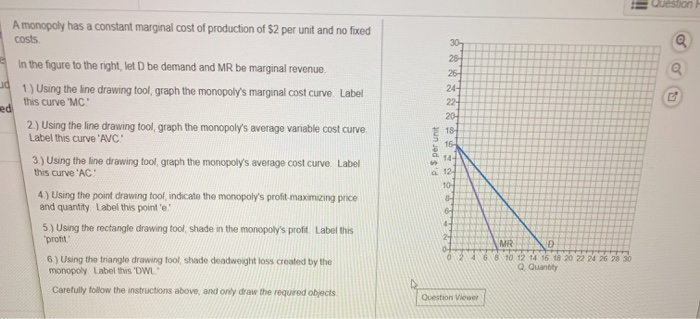Amonopoly has a constant marginal cost of production of \$2 per unit and no foed costs In the figure to the right, let D be demand and MR be marginal revenue ed 1.) Using the line drawing tool, graph the monopoly's marginal cost curve Label this curve 'MC 2) Using the line drawing tool graph the monopoly's average variable cost curve Label this curve 'AVC 3.) Using the line drawing tool graph the monopoly's average cost curve Label this curve...

• ### The graph on the right shows a labor supply and labor demand curve. Illustrate the impact...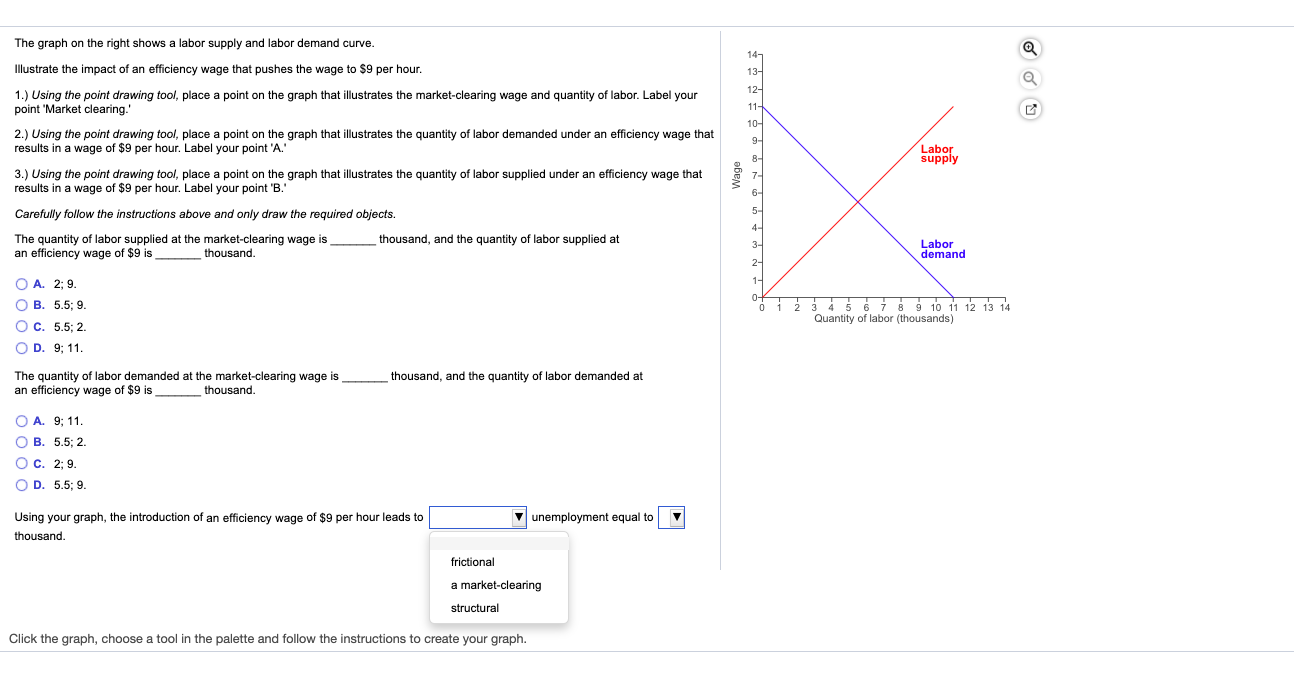The graph on the right shows a labor supply and labor demand curve. Illustrate the impact of an efficiency wage that pushes the wage to \$9 per hour. 1.) Using the point drawing tool, place a point on the graph that illustrates the market-clearing wage and quantity of labor. Label your point Market clearing.' 2.) Using the point drawing tool, place a point on the graph that illustrates the quantity of labor demanded under an efficiency wage that results in...

• ### The graph shows the supply curve of no-name soda. The government has imposed a sales tax...The graph shows the supply curve of no-name soda. The government has imposed a sales tax of \$2 per case on no-name soda. Price (dollars per case) The sellers of no-name soda end up paying the entire tax. @ O3 Draw and label the demand curve for no-name soda. The more the demand, O A. inelastic; the larger is the amount of the tax paid by sellers O B. elastic; the larger is the amount of the tax paid by...

• ### Federal funds rate (percent per year) The graph shows the demand curve for bank reserves, RD....

Federal funds rate (percent per year) The graph shows the demand curve for bank reserves, RD. The current quantity of reserves supplied is \$20 billion. 7 Draw a point on the curve that shows the federal funds rate when the quantity of reserves supplied is \$20 billion. Label it 1 6- 5- t 4 percent a year The Fed wants to set the federal funds rate Draw a supply of reserves curve that achieves the target. Label it Draw a...

• ### Can someone please explain C. Role of Government 1. Draw a supply and demand graph with a binding price ceiling. Label...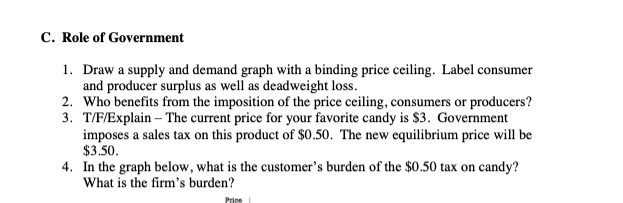Can someone please explain C. Role of Government 1. Draw a supply and demand graph with a binding price ceiling. Label consumer and producer surplus as well as deadweight loss 2. Who benefits from the imposition of the price ceiling 3. T/F/Explain The current price for your favorite candy is \$3. Government imposes a sales tax on this product of \$0.50. The new equilibrium price will be \$3.50 4. In the graph below, what is the customer's burden of the...

• ### A monopoly has a constant marginal cost of production of \$4 per unit and no fixed...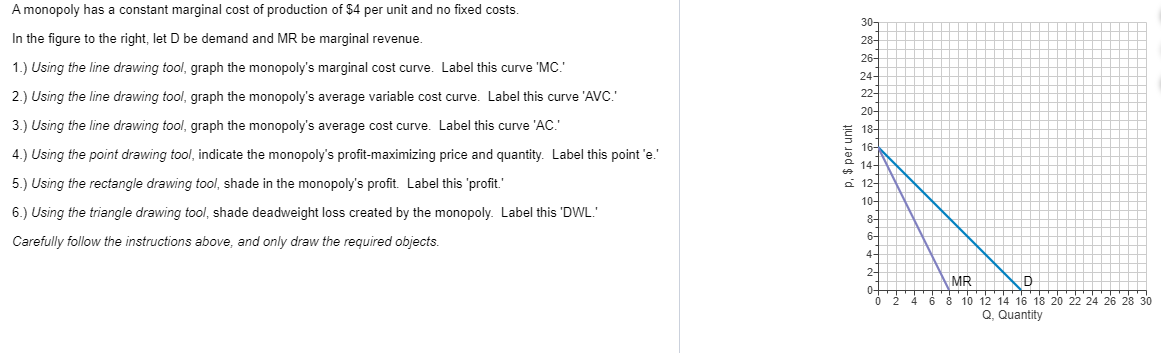A monopoly has a constant marginal cost of production of \$4 per unit and no fixed costs. In the figure to the right, let D be demand and MR be marginal revenue. 1.) Using the line drawing tool graph the monopoly's marginal cost curve. Label this curve 'MC! 2.) Using the line drawing tool, graph the monopoly's average variable cost curve. Label this curve 'AVC.' 3.) Using the line drawing tool, graph the monopoly's average cost curve. Label this curve...

• ### Price (dollars per case) The graph shows the supply curve of no-name soda. The government has imposed a sales tax of \$2...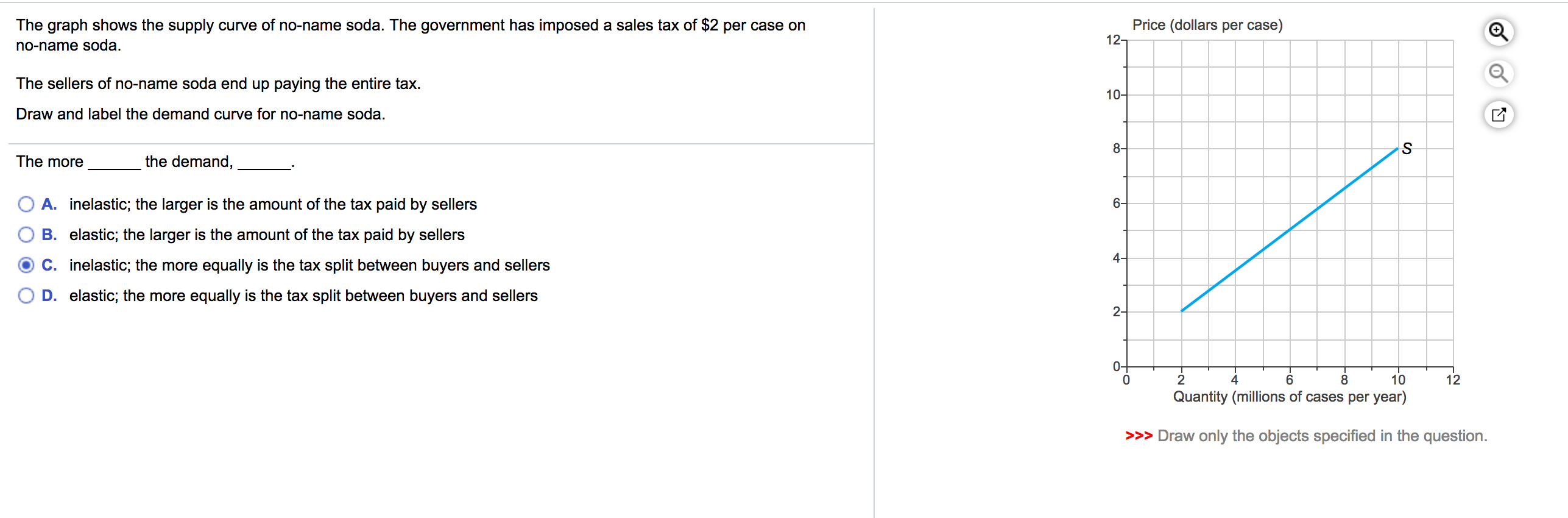Price (dollars per case) The graph shows the supply curve of no-name soda. The government has imposed a sales tax of \$2 per case on no-name soda. The sellers of no-name soda end up paying the entire tax. Draw and label the demand curve for no-name soda. The more the demand, O A. inelastic; the larger is the amount of the tax paid by sellers O B. elastic; the larger is the amount of the tax paid by sellers O...

• ### A monopoly has a constant marginal cost of production of \$2 per unit and no fixed...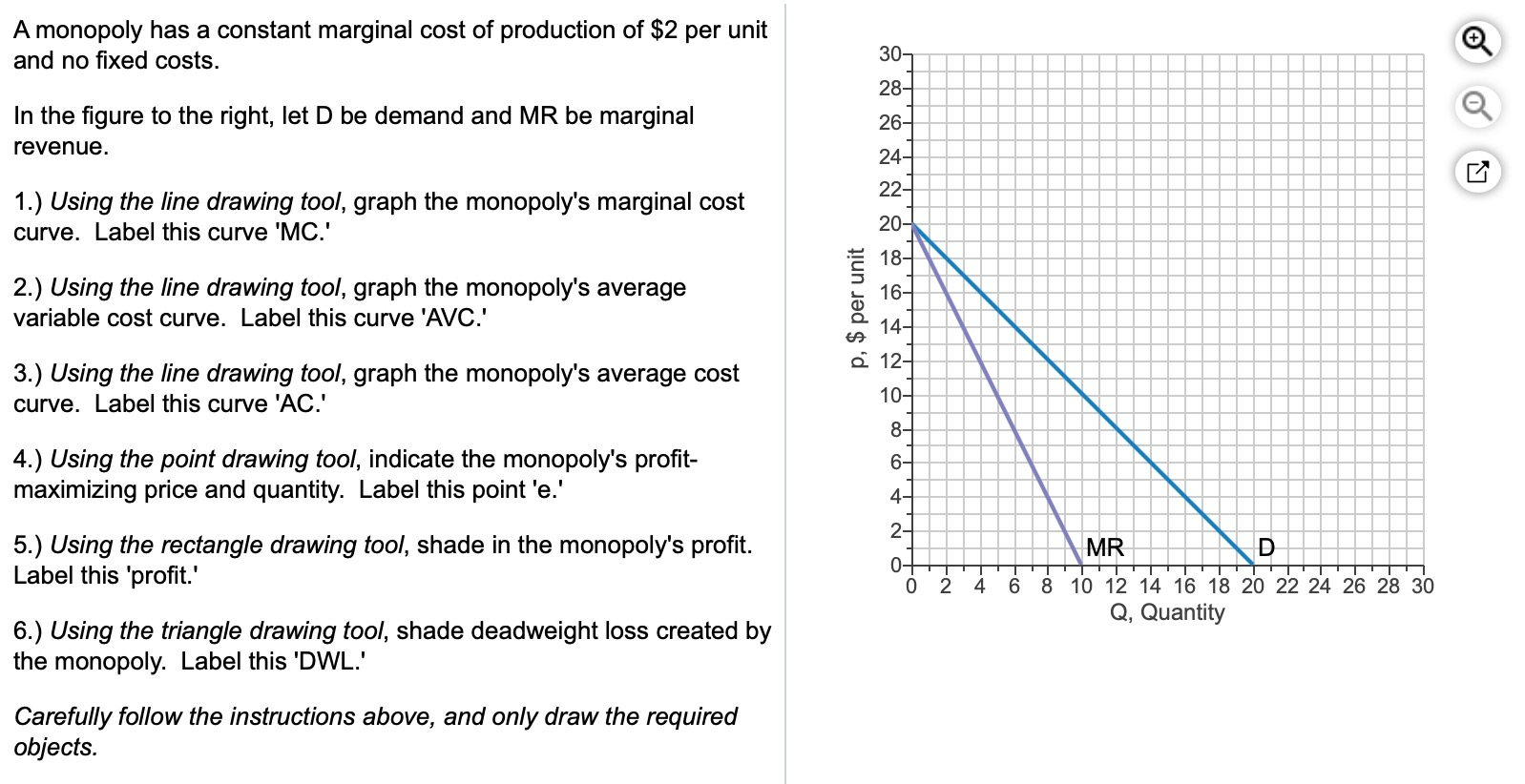A monopoly has a constant marginal cost of production of \$2 per unit and no fixed costs. In the figure to the right, let D be demand and MR be marginal revenue. TTT 1.) Using the line drawing tool, graph the monopoly's marginal cost curve. Label this curve 'MC.' 2.) Using the line drawing tool, graph the monopoly's average variable cost curve. Label this curve 'AVC.' p, \$ per unit 3.) Using the line drawing tool, graph the monopoly's average...

Need Online Homework Help?